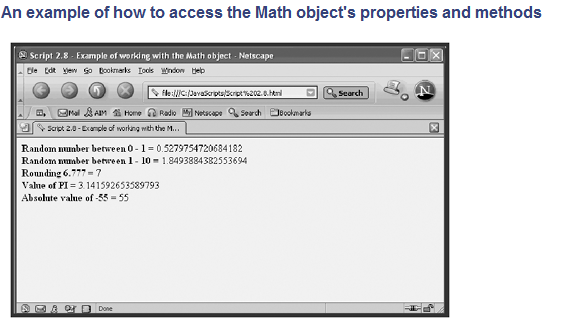Home » Online Computer Education » Learn Java Script, The Math Object, Lesson No. 18

# Learn Java Script, The Math Object, Lesson No. 18

Having introduced you to the String object, it seems only proper at this point in the discussion that I talk a little about the Math object as well. This object is created automatically in every JavaScript and JScript, so you do not have to create an instance of it as you do with the String object. You can simply refer to the Math object when you need to. Like other objects, the Math object has properties and methods associated with it.

The Math object’s properties contain mathematical constants. For example, the Math property PI stores the value of pi. The Math object’s methods contain built-in mathematical functions. There are methods that round numbers up and down, generate random numbers, and return the absolute value of a number.

The following example demonstrates how to use several of the Math object’s properties and methods:

<HTML>
<TITLE>Script 2.8 – Example of working with the Math
object</TITLE>
<BODY>
<SCRIPT LANGUAGE=”JavaScript” TYPE=”Text/JavaScript”>
<!– Start hiding JavaScript statements
//Generate a random number between 0 and 1
document.write(“<B>Random number between 0 – 1 = </B>”, +
Math.random(), “<BR>”);
//Generate a random number between 0 and 10
document.write(“<B>Random number between 0 – 10 = </B>”, +
Math.random() * 10, “<BR>”);
//Rounding a number to the nearest whole number
document.write(“<B>Rounding 6.777 = </B>”,
Math.round(6.777), “<BR>”);
//Getting the value of PI
document.write(“<B>Value of PI = </B>”, Math.PI, “<BR>”);
//Getting the absolute value of a number
document.write(“<B>Absolute value of -55 = </B>”,
Math.abs(-55));
// End hiding JavaScript statements –>
</SCRIPT>
</BODY>
</HTML>
NOTE
You may have noticed that I slipped the <B> and </B> HTML tags into my JavaScripts to add bold formatting to my text.The key when using HTML tags is to keep them within the quotation marks. I could just as easily have inserted any of the other HTML tags within my script using the same technique.
The first three document.write() statements use the Math object’s random() and round() methods. The fourth document.write() statement does not use a Math method. Instead, it accesses the Math PI property as evidenced by the lack of parentheses. The last of the document.write() statements demonstrates the Math abs() method, which returns the absolute value of any given number. Figure 2.5 shows the results of loading this page using Netscape Communicator.The main objective of this website is to provide quality study material to all students (from 1st to 12th class of any board) irrespective of their background as our motto is “Education for Everyone”. It is also a very good platform for teachers who want to share their valuable knowledge.